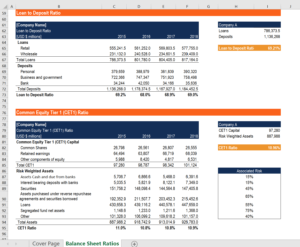# Bank Balance Sheet Ratio Calculator

## Bank Balance Sheet Ratio Calculator

The Bank Balance Sheet Ratio Calculator is a tool that you can use to determine a bank’s financial stability and liquidity using items found on a balance sheet. Using the inputs, the calculator will produce ratios that are important for the analysis of a bank’s balance sheet.

This template includes the following ratios:

• Loan to Deposit Ratio
• Common Equity Tier 1 (CET1) Ratio
• Leverage Ratio

Here is a quick preview of CFI’s Bank Balance Sheet Ratio Calculator:### Loan to Deposit Ratio (LDR)

The loan to deposit ratio is used to determine a bank’s liquidity by comparing the total amount of loan outstanding to deposits stored in a bank.

The formula for the loan to deposit ratio is:

Loan to Deposit = Total Loans / Total Deposits

A high LDR means a bank has a relatively higher amount of capital in loans than deposits. While having a high LDR means a bank has good liquidity, it could also imply that they are not utilizing their capital efficiently. An exceptionally low LDR means the bank is not using the deposits (capital) to generate profit through loans.

### Common Equity Tier 1 (CET1) Ratio

The Common Equity Tier 1 (CET1) Ratio is a requirement set by Basel III. This ratio measures the financial strength of a bank. This is done by comparing a bank’s core capital to its risk-weighted assets.

The formula for the CET1 ratio is:

CET1 Ratio = Common Equity Tier 1 Capital / Risk Weighted Assets

Basel III requires all banks to have a ratio of above 4.5%. This helps protect a bank as if assets suddenly lose value, there is still enough liquid capital to cover losses.

### Leverage Ratio

The leverage ratio is another requirement introduced by Basel III. Similar to the CET1 ratio, it also compares capital to assets. However, for the leverage ratio, the asset is not weighted by risk and tier 1 capital is used.

The formula for the leverage ratio is:

Leverage Ratio: Tier 1 Capital / Total Assets (Exposure)

Basel III set a minimum leverage ratio requirement of 3%. This helps regulators ensure a bank has enough high-quality capital to cover its exposures.

### More Free Templates

For more resources, check out CFI’s business templates library to download numerous free Excel modelling, PowerPoint presentation, and Word document templates.

### Financial Analyst Certification

Become a certified Financial Modeling and Valuation Analyst (FMVA)® by completing CFI’s online financial modeling classes and training program!